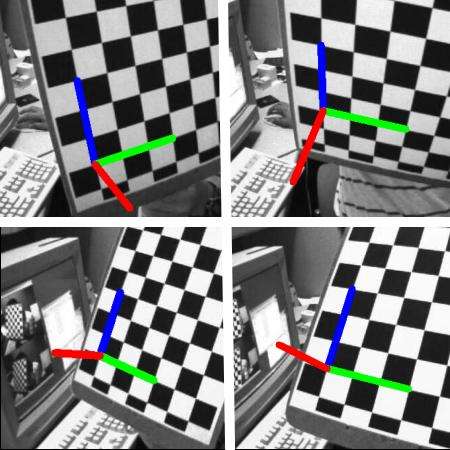# 姿态估计¶

### 基础¶

import numpy as np
import cv2 as cv
import glob
# 加载先前保存的数据
mtx, dist, _, _ = [X[i] for i in ('mtx','dist','rvecs','tvecs')]


def draw(img, corners, imgpts):
corner = tuple(corners.ravel())
img = cv.line(img, corner, tuple(imgpts.ravel()), (255,0,0), 5)
img = cv.line(img, corner, tuple(imgpts.ravel()), (0,255,0), 5)
img = cv.line(img, corner, tuple(imgpts.ravel()), (0,0,255), 5)
return img


criteria = (cv.TERM_CRITERIA_EPS + cv.TERM_CRITERIA_MAX_ITER, 30, 0.001)
objp = np.zeros((6*7,3), np.float32)
objp[:,:2] = np.mgrid[0:7,0:6].T.reshape(-1,2)
axis = np.float32([[3,0,0], [0,3,0], [0,0,-3]]).reshape(-1,3)


for fname in glob.glob('left*.jpg'):
gray = cv.cvtColor(img,cv.COLOR_BGR2GRAY)
ret, corners = cv.findChessboardCorners(gray, (7,6),None)
if ret == True:
corners2 = cv.cornerSubPix(gray,corners,(11,11),(-1,-1),criteria)
# 找到旋转和平移矢量。
ret,rvecs, tvecs = cv.solvePnP(objp, corners2, mtx, dist)
# 将3D点投影到图像平面
imgpts, jac = cv.projectPoints(axis, rvecs, tvecs, mtx, dist)
img = draw(img,corners2,imgpts)
cv.imshow('img',img)
k = cv.waitKey(0) & 0xFF
if k == ord('s'):
cv.imwrite(fname[:6]+'.png', img)
cv.destroyAllWindows()#### 绘制立方体¶

def draw(img, corners, imgpts):
imgpts = np.int32(imgpts).reshape(-1,2)
# 用绿色绘制底层
img = cv.drawContours(img, [imgpts[:4]],-1,(0,255,0),-3)
# 用蓝色绘制高
for i,j in zip(range(4),range(4,8)):
img = cv.line(img, tuple(imgpts[i]), tuple(imgpts[j]),(255),3)
# 用红色绘制顶层
img = cv.drawContours(img, [imgpts[4:]],-1,(0,0,255),3)
return img


axis = np.float32([[0,0,0], [0,3,0], [3,3,0], [3,0,0],                    [0,0,-3],[0,3,-3],[3,3,-3],[3,0,-3] ])